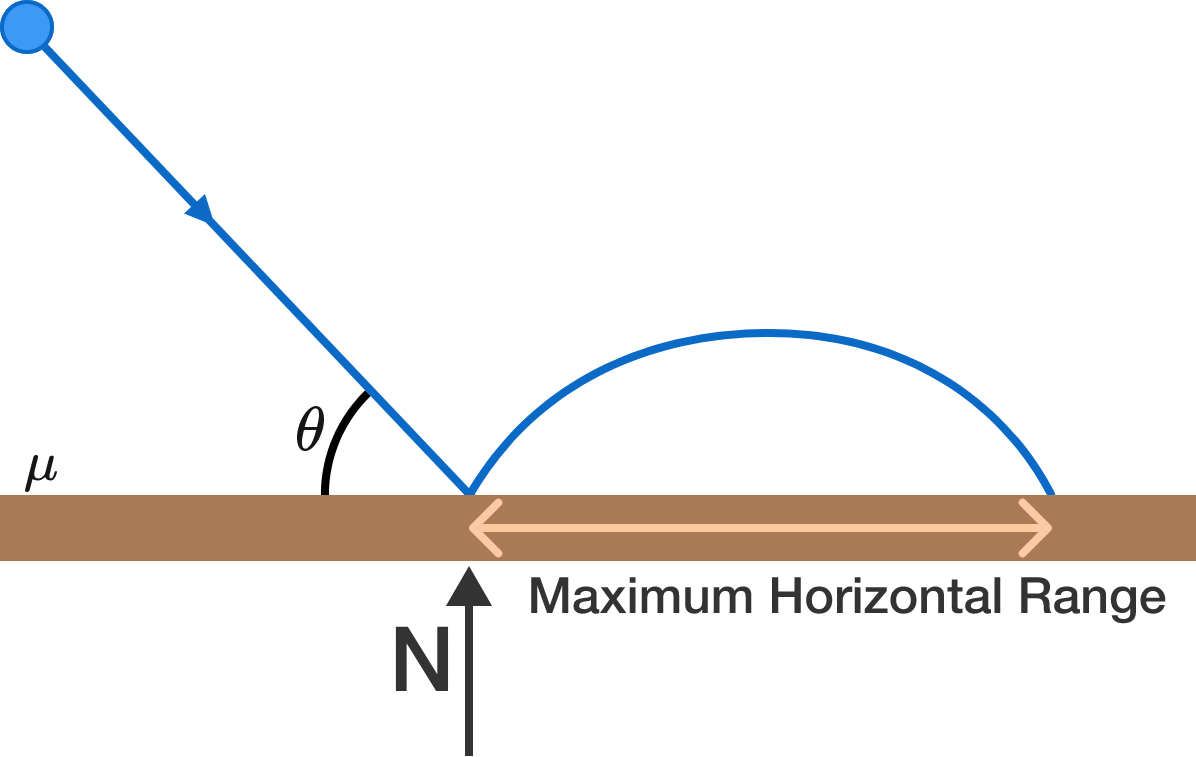# Friction in Elastic collision?A small spherical ball undergoes an elastic collision with a rough horizontal surface. Before the collision, it is moving at an angle of $\theta$ with the horizontal. Assume that friction $f=\mu N$ during the contact period, where $N$ is the normal reaction and $\mu$ is the coefficient of friction.

Find the value of $\theta$ (in degrees) to the nearest integer such that the horizontal range of the ball after hitting the surface is maximized, given $\mu=\frac{\sqrt{3}}{2}$.

×